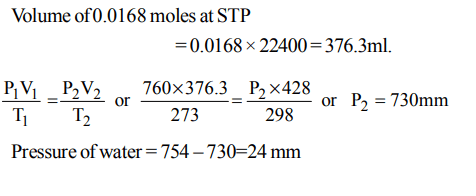## States of Matter Questions and Answers Part-4

1. The compressibility factor for an ideal gas is
a) 1.5
b) 1.0
c) 2.0
d) $\propto$

Explanation: Ideal gas strictly follows PV= nRT, Z = $\frac{PV}{nRT}$ = 1

2. A gas will approach ideal behaviour at
a) low temperature and low pressure
b) low temperature and high pressure
c) high temperature and low pressure
d) high temperature and high pressure

Explanation: Ideal behaviour at low P and high temperature. The larger the volume the lesser are the interactions, higher the temperature the more is KE the lesser are interactions between the molecules.

3. The compressibility of a gas is less than unity at STP. Therefore
a) $V_{m}>22.4 litres$
b) $V_{m}<22.4 litres$
c) $V_{m}=22.4 litres$
d) $V_{m}=44.8 litres$

Explanation: Z = $\frac{PV}{nRT}$ < 1
nRT > PV
1*0.0821*273 > 1*V or V < 22.4

4. An ideal gas will have maximum density when
a) P = 0.5 atm, T = 600 K
b) P = 2 atm, T = 150 K
c) P = 1 atm, T = 300 K
d) P = 1 atm, T = 500 K

Explanation: Higher P lower T greater the density

5. 120 g of an ideal gas of molecular weight $40 g mol^{-1}$ are confined to a volume of 20 L at 400 K. Using R=0.0821 L atm $K^{-1}mol^{-1}$ , the pressure of the gas is
a) 4.90 atm
b) 4.92 atm
c) 5.02 atm
d) 4.96 atm

Explanation:6. The volume of 0.0168 mol of $O_{2}$ obtained by decomposition of $KClO_{3}$ and collected by displacement of water is 428 ml at a pressure of 754 mm Hg at 250 C. The pressure of water vapour at 250 C is
a) 18 mm Hg
b) 20 mm Hg
c) 22 mm Hg
d) 24 mm Hg

Explanation:7. Pressure of a mixture of 4 g of $O_{2}$ and 2 g of $H_{2}$ confined in a bulb of 1 litre at 00 C is
a) 25.215 atm
b) 31.205 atm
c) 45.215 atm
d) 15.210 atm

Explanation:8. Same mass of $CH_{4}and H_{2}$   is taken in container, The partial pressure caused by $H_{2}$ is
a) $\frac{8}{9}$
b) $\frac{1}{9}$
c) $\frac{1}{2}$
d) 1

Explanation:9. There are $6.02* 10^{22}$   molecules each of $N_{2},O_{2} andH_{2}$   which are mixed together at 760 mm and 273 K. The mass of the mixture in grams is
a) 6.02
b) 4.12
c) 3.09
d) 6.2